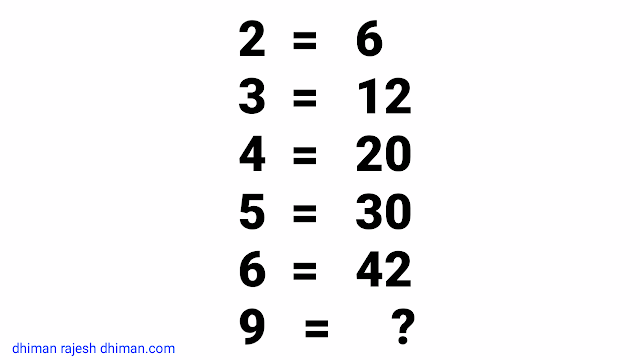reasoning (43) Maths (40) Home (8) integration (6)

## HOW TO SOLVE IMPOSSIBLE AND HARD PUZZLES

Hello Friends Most welcome to this post of my Blog . In this post We are going to discuss some of the puzzles, quizzes , riddles, challenges and brain teaser which are viral in social media such as Face book ,whats app, twitter and Google + etc. which are based on Mathematics directly or indirectly . You also have seen such types of problems mentioned 99.9% Fail , Solve if you are genius like this...

Let us try to discuss and solve some of these Puzzles one by one.

## Puzzle #1

If
2 = 6
3 = 12
4 = 20
5 = 30
6 = 42
then 9 = ?
In this problem two steps are missing
7= ?
8 = ?
and directly asked how 9 = ?HOW TO SOLVE IMPOSSIBLE AND HARD PUZZLES
How 2 is related to 6 , in the same manners 3 is related to 12 and 4 is also related to 20  and so on.

If we  multiply   2 with its successor ( the immediate  next number) i.e. 3 then we get 2×3 = 6,  Similarly  multiply 3 with its successor 4 we get 3 ×4 =12 ,4×5 =20  and 5 ×6 =30 ,6×7=42 and ( and the missed numbers 7×8=56,8×9=72 )

and finally we have a formula for this quiz which is (a)×(a+1) ,where  "a" is given number.

The required answer    is 9 ×10 = 90

Read  " How to Multiply Two numbers in quick Time " to  save your time in calculations

Now let us move to little bit harder problem below

## Puzzle #2

Look at this problem , To solve such types of puzzles ,1st of all do not count "+" ,"-" or any mathematical sign as it appears. i.e in such puzzles + does not means addition and also  - do not means subtraction. we our self have to make a formula to satisfies given results and conditions we have to perform such mathematical  operations whose result must be the number given in the right side .
So if we add 20+20 which is 40 and then divide it with 10 we shall get 4,

Similarly So if we add 15+15 which is 30 and then divide it with 10 we shall get 3,
so at last if we add 30+15 which is 45 and then divide it with 10 we shall get   4.5  as answer.

So the formula for this quiz is a+b =  (a+b)/10
---------------------------------------------------- ------------------------------------------------ -----------------------

## Puzzle # 3

Here it is given that 6+9 =61
5+8 = 46
4+7 = 33
3+6 = 22
2+1 = Missing
1+4 = ?
Most of peoples forget to understand that one step has been left intentionally to  make us fool or we can say it is the part of their strategy.

### Step 1

If we  Multiply   6 ×9 then it is equal to 54 but not equal to the given result 61,

### Step 2

If we add successor of 1st term to the result just obtained then it  is equal to 54+(6+1) = 61. yes it is , but is it true for  next step.

5×8=40 , adding 1st term's successor to the result just obtained i.e 6
5×8+(5+1)=40+6=46, yes it works

Now let us verify for remaining steps
4×7+(4+1) = 28+5 =33 , ok
3×6+(3+1)=18+4=22 ,  it is ok for all given results
So we have discovered the trends
1 Multiply    both the given numbers
2 Add the successor of the 1st number to the result obtained in step 1 ,and we have our answers every time in this puzzles,
.
.
So using the formula so discovered the required term must be  1×4+(1+1)=4+2 =6

Now the formula for this puzzle is a+b = (a×b)+(a+1)

## Conclusion

Thanks for devoting your valuable time for this post  "How   to solve various puzzles ,Quizzes ,  Brain Teasers and challenges " of my blog . If you liked this this blog/post,  Do Follow me on my blog and share this post with your friends . We shall meet again   in next post with solutions of most interesting and mind blowing puzzles ,till then Good Bye.

## Magical   Maths

Share:

1.1.